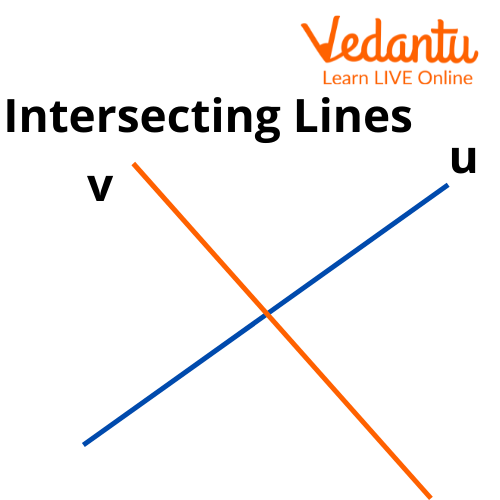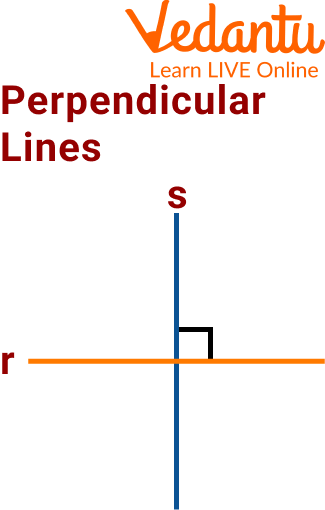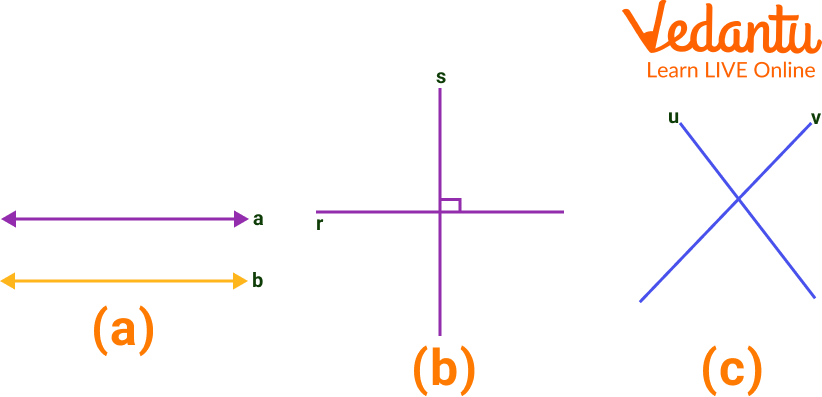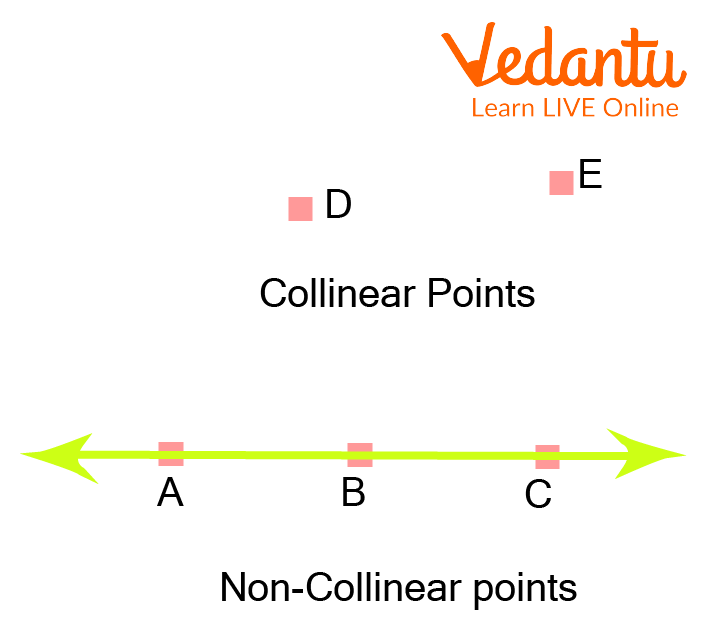Courses
Courses for Kids
Free study material
Offline Centres
More

# Point, Line, and Plane - The Three Undefined Terms in GeometryLast updated date: 24th Nov 2023
Total views: 130.2k
Views today: 1.30k## Introduction to the Basic Concepts of Geometry

To begin the study of geometry, it is necessary to know the most basic concept of geometry. However, they cannot be defined. Fortunately, we have innate ideas about these concepts.

Surprisingly, there are three undefined terms of geometry. These three things are called undefined terms because in geometry they do not require a formal definition. They serve as the cornerstones for establishing other terms and theorems. These words themselves are so basic that they are considered true without formally defining them.

They are point, line, and plane. These basic geometrical ideas are defined below.

## Point, Line, and Plane

The point, line, and plane are basic geometrical ideas and are not defined. They are considered "primitive concepts" and are the basis of Geometry. We will consider space as a set of points and then, we will be able to give an idea of ​​what a point, line, and plane are:

• A point is the smallest object in space, it has no dimension (neither length nor width).

• Straight is a line that "does not bend". It has one dimension (it has length, but no width).

• The surface on which points and lines can be drawn is called a plane. It is two-dimensional (length and width).

## Collinear and Non-Collinear Points

Points that are on the same straight line are referred to as collinear points. These kinds of lines have two points in common. They must lie on the same straight line, even if they don't have to be coplanar.

Non-collinear points are three or more points that do not all lie on the same straight line. They are considered non-collinear points if any one of the points among them is not on the same line.

## Types of Straight Lines According to the Position Between them

1. Parallel lines are in the same plane and maintain a certain distance from each other, but they never cross or touch at any point.

An example of parallel lines would be train tracks, even though they seem to touch in the distance. It is because of that feeling that the train tracks are getting closer, but it is not true, besides, infinity is not a point, so saying that they touch at infinity is a curious way of saying that they never touch.Parallel Lines

2. intersect at a point. When cut, they divide the plane into 4 regions, that is why we say that they form 4 angles. They have one point in common.Intersecting Lines

3. Perpendicular lines are a particular case of intersecting lines, in addition to intersecting at a point and forming four right angles (angle of 90 degrees).Perpendicular Lines

## Solved Examples

Q 1. Find the correct types of lines from the figure given below.Perpendicular, Parallel and Intersecting Lines

Sol: (a) A pair of parallel lines.

(b) A pair of intersecting or non-parallel lines.

(c) An illustration of perpendicular lines.

Q 2. Identify the collinear points and non-collinear points in the figure given below.Collinear and Non-collinear Points

Sol: Collinear points are A, B, and C as they all lie on the same straight line. Points D and E are not collinear because they do not lie on the same line.

Q 3. Define parallel lines.

Ans: Parallel lines are coplanar lines. They do not intersect at any point. Although they seem to be intersecting at infinity it is only a curious way to say that they do not intersect at any point.

Q 4. What is a plane in geometry?

Ans: A plane is a flat, two-dimensional surface that consists of all the points that form a straight line by joining each other.

Q 5. Define a straight line and what are coplanar points?

Ans: A straight line is formed by joining two points on a plane, it has length but no width. Coplanar points are the points that lie on the same plane.

## Practice Questions

Q 1 Name the undefined terms of geometry.

Ans: Point, Line, Plane

Q 2 Choose the correct statement.

(a) A point has length, breadth, and width

(b) Collinear points do not lie on the same row

(c) A point is represented by a dot.

Ans: (c).

Q 3 Differentiate between parallel lines and intersecting lines.

Ans: Parallel lines do not intersect and intersecting lines intersect at certain points and have one point in common.

Q 4 Why do we say that parallel lines meet at infinity?

Ans: They only seem to meet, in reality, they do not.

Q 5 Define coplanar points and collinear points.

Ans: Coplanar points are those which lie on the same plane whereas collinear points lie on the same line and have two points in common.

## Summary

We have learned the three undefined terms of geometry. Undefined terms are those terms that do not require a formal definition. The three terms are point, line, and plane. A point is just a point and is labeled with a capital letter. An endlessly long, straight mark is known as a line and is labeled with two capital letters that represent two points on the line. A plane is a flat surface that never ends in any direction and is labeled with a capital letter along with the word "plane."

## FAQs on Point, Line, and Plane - The Three Undefined Terms in Geometry

1. What are the undefined terms of plane geometry?

In geometry, formal definitions are formed using other defined words or terms. There are, however, three words in geometry that are not formally defined. These words are point, line, and plane, and are referred to as the "three undefined terms of geometry".

2. How many types of lines are there? What is a real-world example of a line?

A geometric figure that can travel in both directions is a line. There are infinitely many points that make up a line. It has no beginning and no end and is one-dimensional. There are two types of lines: straight and curved. Parallel, intersecting, and perpendicular lines are further types of lines.

A pencil, a baseball bat, and other everyday objects are examples of line segments

3. Why is the study of point, plane, and line important?

Being the basic concepts of geometry, their study is crucial to understanding most concepts of geometry. These three are the starting place of geometry. Their not-so-clear definition gives the proper basics for most of the official definitions in geometry.